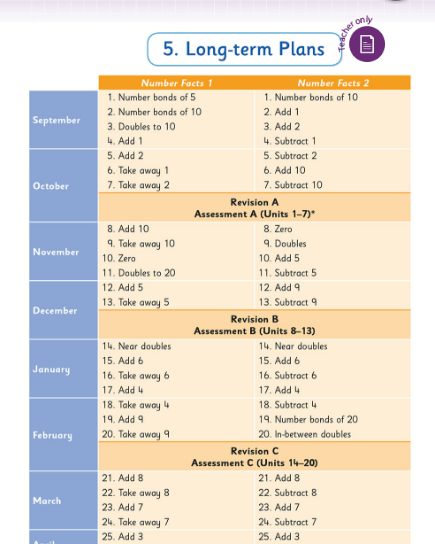# Thinking Strategies for Multiplication and Division Number Facts

## Thinking Strategies for Multiplication and Division Number Facts

Category : Uncategorized

### What are number facts?

Number facts are the basic number facts that, it is hoped, children could recall instantly, so as to improve their ability to compute mentally and use written algorithms. Traditionally referred to as tables, the multiplication and division number facts typically include all the multiplication facts up to 10 x 10 and their inverse division sentences.

Some of the big ideas about number facts:

• Some facts are easier than others to recall – which ones, do you think?
• The easier facts can be used to calculate other facts – which ones, do you think?
• The same fact can be calculated using various approaches – these approaches are often referred to as thinking strategies – see more below.
• Using thinking strategies means that the children can apply the understanding, to facts beyond the traditional limits of “tables”.

### What are thinking strategies?

A thinking strategy is a way to think about a process to arrive efficiently at an answer. For example, if asked to multiply a number by 2, one could double the number. Doubling is a very effective thinking strategy for the multiplication facts of 2, 4 and 8, as can be seen in the video below.

Halving is the opposite to doubling. And halving is a very effective thinking strategy to use for the multiplication facts of 5; if asked to multiply a number by 5, one could think of 10 times the number and then halve that amount (see below).

The Operation Maths  and Number Facts books for third and fourth classes repeatedly emphasise (among other thinking strategies) the strategy of doubling and halving known facts to derive unknown facts, eg through doubling I can work out 2 times, 4 times and 8 times a number; if I know 10 times the number I can work out 5 times, etc.From Operation Maths 3, possible thinking strategies for 2x, 5x, 10x.

The 100 dots grids on the inside back covers of Operation Maths 3 and 4 and Number Facts 3 and 4 can be extremely useful for the pupils to model various arrangements/arrays, while the teacher can use the Operation Maths 100 square eManipulative to replicate (and label) the children’s arrangements on the IWB.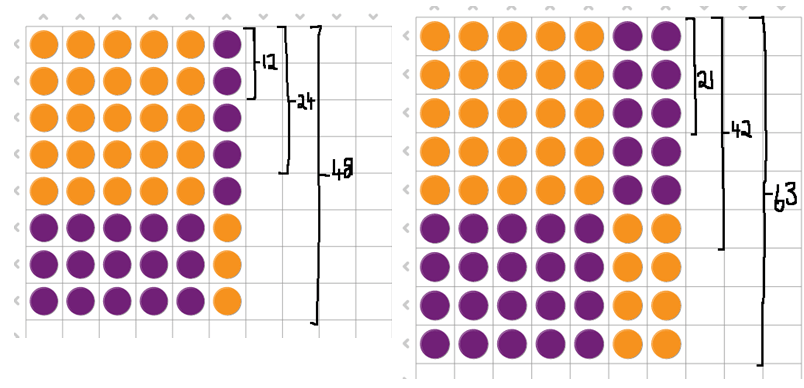Using doubling to model 2 x 6, “2 rows of 6”, 4 x 6, 8 x 6 (left) and trebling to model 3 x 7, 6 x 7, 9 x 7 (right)

Furthermore, multiplication and division are taught together throughout the Operation Maths series, so that, rather than compartmentalising each operation, the children develop a better understanding of how both concepts relate to each other. In this way, the basic division facts are easier to acquire, as they are understood to be the inverse of the more familiar multiplication facts. However, it is important that within each group of facts, the children explore the multiplication facts first; the better their understanding of these, the more likely they are understand the inverse division facts. Indeed, “think multiplication” is in itself, a thinking strategy for the division facts (see video below).

Traditionally, learning “tables” had been by rote, but current research suggests that this is ineffective for the majority of children. In contrast, children should be taught to visualise numbers and to use concrete materials, images and thinking strategies to use what they know to solve what they do not know. Below are examples of some useful thinking strategies for the basic multiplication and division facts (taken from Number Facts 3 & 4, Edco, 2018)There can often be different ways to think about the same fact (or groups of facts), and the children should always be encouraged both to identify alternative approaches and to choose their preferred strategy. For example, consider 5 x 9:

5 times is half of 10 times: 10 × 9 = 90, so 5 × 9 = half of 90 = 45
9 times is one set less than 10 times: 10 × 5 = 50, so 9 × 5 = 50 − 5 = 45
9 times is treble 3 times: 3 × 5 = 15, so 9 × 5 = treble 15 = 45

Once the children understand how to arrive at an answer via a thinking strategy, they can then apply this thinking strategy to more complex calculations that are beyond the traditional 10 x 10 ceiling of “tables”; for example if I understand 5 times any number is half 10 times the number, then I can use this to mentally calculate 5 x 18, 5 x 26 etc (see more on this below).

### Computational Fluency:

‘Fluency requires the children to be accurate, efficient and flexible.’ (Russell, 2000).

The primary aim of both the Operation Maths and Number Facts series (see more information on Number Facts below) is to enable the children to become computationally fluent. To achieve computational fluency, the children must be accurate, efficient and flexible:

• Accurate: the children must arrive at the correct answer, e.g. 6 x 8  =48.
• Efficient: the children must calculate the answer in an efficiently. A child who produces an answer of 48 in response to the question 6 × 8 by counting in jumps of six or eight may be accurate but is not efficient.
• Flexible: children must be able to visualise and mentally manipulate numbers in order to see how they might be broken down and recombined to get an accurate and efficient answer (as shown with the various ways to consider 6 x 8 below).Thus, flexibility is the key to fluency. A child who only knows that 6 x 8 = 48 becasue they have memorized that fact, is missing out on all the various possible connections between those numbers, subsequently hampering future connection-building. In contrast, a child who is flexible with number facts is one with a well-developed number sense, who can see the connections both between and within numbers, i.e. they can partition and/or combine numbers into more compatible (friendly) amounts and can apply their strategies to numbers beyond those they have dealt with. Therefore, a thinking strategies approach will not only be effective for aiding understanding and recall of the basic facts up to 10 x 10, a thinking strategies approach can enable children to apply these mental computation skills to numbers beyond this traditional ceiling, as shown below.From Number Facts 4

### The Number Facts Series from Edco

Number Facts is latest addition to the Edco Primary Maths stable, and it is a series of activity books designed to foster fluency in number facts for primary school children from First Class. The series features an innovative approach to the acquisition of basic number facts, and, like Operation Maths, teaches children to understand, not just do, maths.In contrast to the more traditional drill-and-practice workbooks, which just test whether the answer is known, Number Facts teaches children to visualise numbers pictorially and to use these images and thinking strategies to become more adept at manipulating numbers. The specific focus of Number Facts will be to develop children’s thinking strategies and apply these to the basic number facts in such a way as to promote the child’s ability to visualise and recall these facts, thereby achieving fluency.

Both this rationale, and the suggested teaching approaches to the teaching of the basic multiplication and division facts for third and fourth classes, are clearly outlined in the Teachers Resource Book (TRB) which accompanies the series, and which is downloadable here. This TRB also includes a Long Term Plan for both third and fourth classes (see extract below), outlining a logical progression for the various fact groups throughout the school year. To view sample pages from the pupils Number Facts books please click here. Sample copies of all the books are also available from your local Edco reps.## Thinking Strategies for Addition and Subtraction Number Facts

Category : Uncategorized

### What are number facts?

Number facts are the basic number facts that, it is hoped, children could recall instantly, so as to improve their ability to compute mentally and use written algorithms. Traditionally referred to as tables, the addition and subtraction number facts typically include all the addition facts up to 10 + 10 and their inverse subtraction sentences.

Some of the big ideas about number facts:

• Some facts are easier than others to recall – which ones, do you think?
• The easier facts can be used to calculate other facts – which ones, do you think?
• The same fact can be calculated using various approaches – these approaches are often referred to as thinking strategies – see more below.
• Using thinking strategies means that the children can apply the understanding, to facts beyond the traditional limits of “tables”.

### What are thinking strategies?

A thinking strategy is a way to think about a process to arrive efficiently at an answer. For example, if asked to add 9 to a number, one could think of moving 1 from the other addend to the 9 so as to make a 10, which therefore becomes an easier calculation (see below)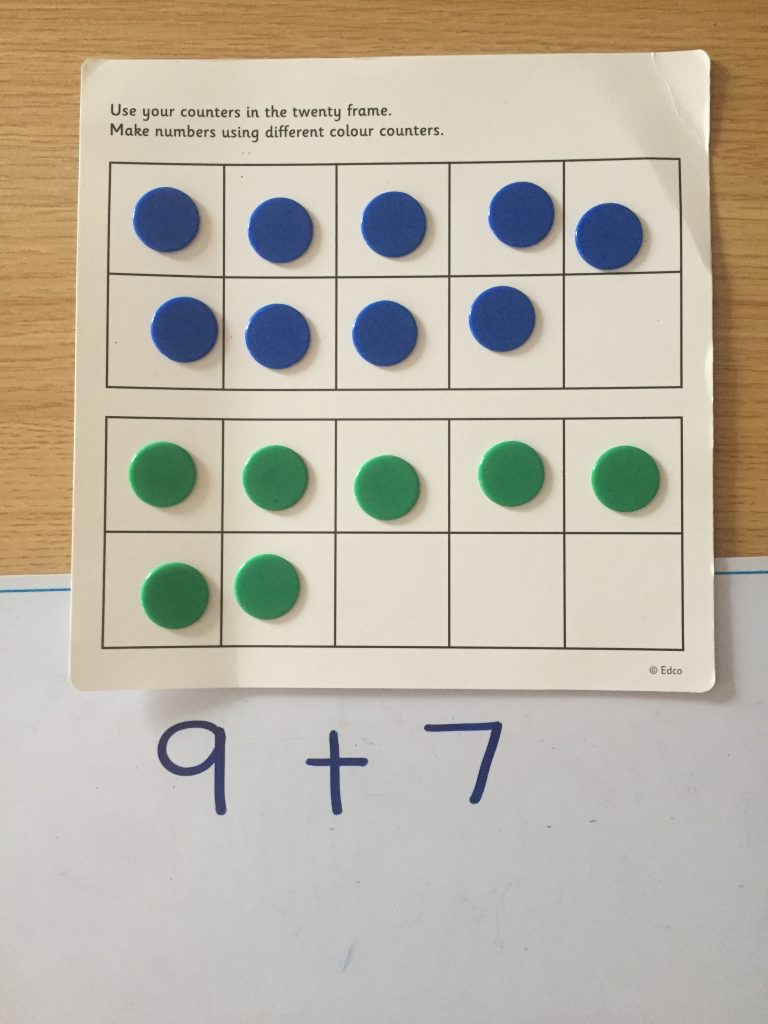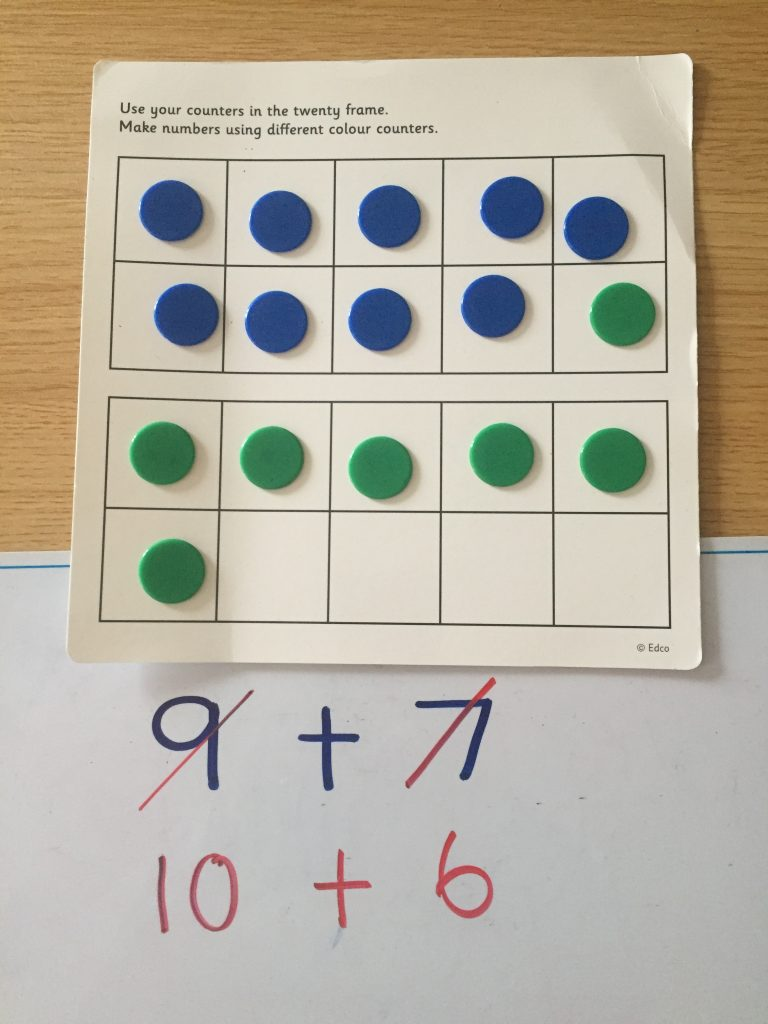The Operation Maths books for first and second classes emphasise three specific thinking strategies throughout: counting on from the biggest number, using doubles and near doubles and using the number bonds for ten (see image below). The doubles facts and bonds of ten are also included on the pull-out flap at the back cover to the pupils books, both for quick reference and to emphasise their importance.From Operation Maths 2 At School Book

In the case of doubles, near doubles and bonds of ten, these key sets of number facts tend to be easier for children to understand and recall. These facts also make up a core section of the total addition facts to 10 + 10, as highlighted below on the addition square. When these become known facts, they can then in turn be used to calculate unknown facts (eg if 7 + 3  = 10, then 7 + 4 = 11), thus covering an even greater number of the total addition facts.Furthermore, addition and subtraction are taught together throughout the Operation Maths series, so that, rather than compartmentalising each operation, the children develop a better understanding of how both concepts relate to each other. In this way, the basic subtraction facts are easier to acquire, as they are understood to be the inverse of the more familiar addition facts.

Traditionally, learning “tables” had been by rote, but current research suggests that this is ineffective for the majority of children. In contrast, children should be taught to visualise numbers and to use concrete materials, images and thinking strategies to use what they know to solve what they do not know. Below are examples of some useful thinking strategies for the basic addition and subtraction facts (taken from Number Facts 1 & 2, Edco, 2018)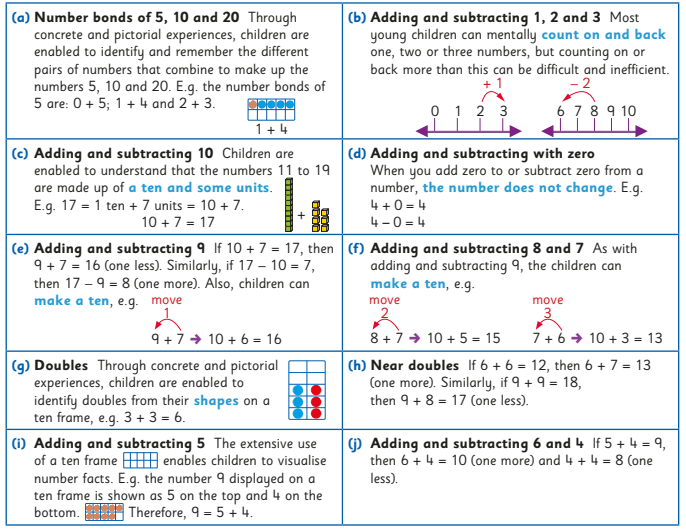From Number Facts 1 & 2

There can often be different ways to think about the same fact (or groups of facts), and the children should always be encouraged both to identify alternative approaches and to choose their preferred strategy. For example:

8 + 6 = (5 + 3) + (5 + 1) = 10 + 4 (make a ten) = 14
8 + 6 = 10 + 4 (move 2 from 6 to 8 to make a ten) = 14
8 + 6 = 7 + 7 (move 1 from 8 to 6 to make a double) = 14

Once the children understand how to arrive at an answer via a thinking strategy, they can then apply this thinking strategy to more complex calculations that are beyond the traditional 10 + 10 ceiling of “tables”; for example if I understand different ways to calculate that 8 + 6 = 14, then I can use these ways to mentally calculate 18 + 6 , 18 + 16 etc.

### Computational Fluency:

‘Fluency requires the children to be accurate, efficient and flexible.’ (Russell, 2000).

The primary aim of both the Operation Maths and Number Facts series (see more information on Number Facts below) is to enable the children to become computationally fluent. To achieve computational fluency, the children must be accurate, efficient and flexible:

• Accurate: the children must arrive at the correct answer, e.g. 8 + 6 = 14.
• Efficient: the children must calculate the answer in an efficiently. A child who produces an answer of 14 in response to the question 8 + 6 by ‘counting all’ (eg have to count up to a total using using counters, fingers, etc.) may be accurate but is not efficient.
• Flexible: children must be able to visualise and mentally manipulate numbers in order to see how they might be broken down and recombined to get an accurate and efficient answer (as shown with the various ways to consider 8 + 6 above).

Thus, flexibility is the key to fluency. A child who only knows that 8 + 6 = 14 becasue they have memorized that fact, is missing out on all the various possible connections between those numbers, subsequently hampering future connection-building. In contrast, a child who is flexible with number facts is one with a well-developed number sense, who can see the connections both between and within numbers, i.e. they can partition and/or combine numbers into more compatible (friendly) amounts and can apply their strategies to numbers beyond those they have dealt with. Thus, a thinking strategies approach will not only be effective for aiding understanding and recall of the basic facts up to 10 + 10, a thinking strategies approach can enable children to apply these mental computation skills to numbers beyond this traditional ceiling e.g. 19+ 5, 29 + 6 etc (see below).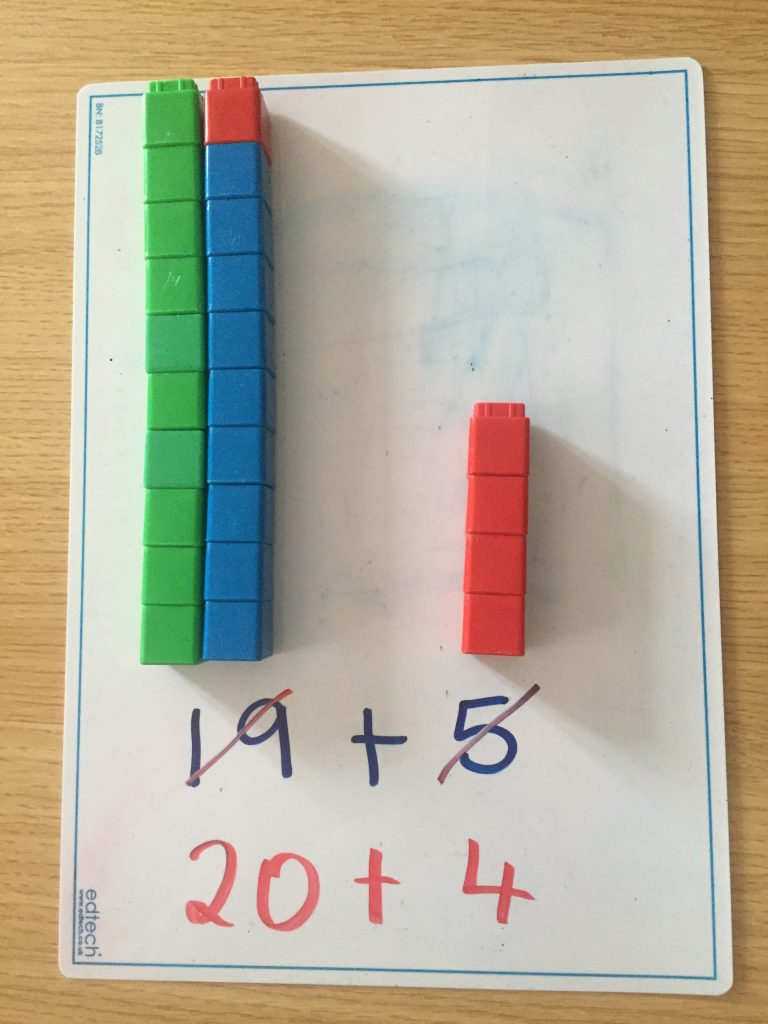### The Number Facts Series from Edco

Number Facts is latest addition to the Edco Primary Maths stable, and it is a series of activity books designed to foster fluency in number facts for primary school children from First Class. The series features an innovative approach to the acquisition of basic number facts, and, like Operation Maths, teaches children to understand, not just do, maths.In contrast to the more traditional drill-and-practice workbooks, which just test whether the answer is known, Number Facts teaches children to visualise numbers pictorially and to use these images and thinking strategies to become more adept at manipulating numbers. The specific focus of Number Facts will be to develop children’s thinking strategies and apply these to the basic number facts in such a way as to promote the child’s ability to visualise and recall these facts, thereby achieving fluency.

Both this rationale, and the suggested teaching approaches to the teaching of the basic addition and subtractions facts for first and second classes, are clearly outlined in the Teachers Resource Book (TRB) which accompanies the series, and which is downloadable here. This TRB also includes a Long Term Plan for both first and second classes (see extract below), outlining a logical progression for the various fact groups throughout the school year. To view sample pages from the pupils Number Facts books please click here. Sample copies of all the books are also available from your local Edco reps.